## Average For SBI PO set 4

1. The average weight of 3 groups of friends of 50, 30 & 20 members respectively, are 50 kg 52 kg and 55 kg. What is the average weight of all the friends taken together?

(a) 49 kg

(b) 58 kg

(c) 51.6 kg

(d) 50.2 kg

(a) 49 kg

Explanation:

Average weight of all (50+30+20) 100 members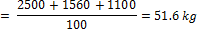2. Abhimanyu goes from his office to his house at the speed of 60 kmph and returns to his office at the speed of 100 kmph. What is the average speed for the entire journey?

(a) 75 kmph

(b) 85 kmph

(c) 65 kmph

(d) none of these

(a) 75 kmph

Explanation:

Since distance is the same hence, use the formula,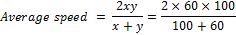= 1200/160=75 knph

3. The average of the age of A, B, C is 25 years, while the average of the age of A, B and D is 28 years. If D is 28 years old, then what is the age of C?

(a) 20 years

(b) 18 years

(c) 25 years

(d) none of these

(d) none of these

Explanation:

Sum of age of A+ B+C = 25 x 3 = 75 years

Also A+B+D= 28 x 3 = 84 years

D-C = 9 years

We are given. D = 28 years, hence C = 19 years

4. The average age of ‘p’ students of a class is ‘a’ years. If the average age of ‘q’ of them is ‘b’ years, then what is the average age of the remaining students?

(a)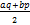(b)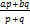(c)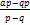(d)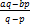(c)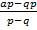Explanation:

Sum of the age of ‘q’ students=ap

Sum of the age of ‘q’ [which are also included in p]= bq

Average age of remaining students (p-q)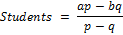5. The average age of all employees of TCS is 35 years. It was later found that age of one of the employee has been taken as 10 years less than his actual age, so the new average after correction, increased by 0.5 years. The no. of employees in TCS are

(a) 12

(b) 20

(c) 15

(d) 18

(b) 20

Explanation:

let there be ‘x’ employees in TCS.

The extra 10 years would be divided amongst ‘x’

Employees and average goes up by 0.5 years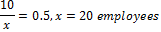Method-2: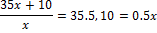And x = 20

[Incorrect sum of age=35x, correct sum of ages=35x + 10 and no. of employees is x]

6. The average height of a group of 12 boys increases by 2 inches when a boy of height 52 inches is replace by another boy. What is the height of the new boy?

(a) 54

(b) 76

(c) 72

(d) 48

(b) 76

Explanation:

Average height increases by 2 inches

Sum of height increases by 2 x 12 = 24 inches

Height of new boy = 52 +24 = 76 inches

7. The average age of members of a family in the year 2000 was 35 years. What is the average age of the family in year 2002?

(a) 36

(b) 34

(c) 37

(d) Cannot be determined

(c) 37

Explanation:

Since the age of all the members would increases by 2, hence the average age would also increases by 2 and hence average age in 2002 would be 35+2= 37 years

8. The average age of Ram, Laxman, Ravan and Vibhishan is 22 years. Vibhishan leaves the group. If Vibhishan was 24 years old, then find the new average of the group

(a) 22.3 years

(b) 21.3 years

(c) 22.1 years

(d) 24 years

(b) 21.3 years

Explanation:

Old average = 22 years

New average would decreases by 2/3 years

New average = 22 – 2/3=64/3=21.33 years

9. On an average, Mukesh scored 85 marks in Hindi, English, Maths and Physics. If the marks of Chemistry are also included, the average becomes 80. How much did Mukesh score in chemistry?

(a) 60

(b) 65

(c) 70

(d) 75

(a) 60

Explanation:

Average marks are decreased by 5, so sum would decreases by 5 x 5=25. Hence marks obtained in Chemistry = 85-25=60

10. The average of 6 numbers is 10. If each of these numbers is divided by 2 and then 1 is subtracted from each of these resultant numbers, then find the new average?

(a) 5

(b) 4

(c) 2

(d) Cannot be determined

(b) 4

Explanation:

Step-I:

The new average would be 10/2=5

[This is because when each no. is divided by same number, say x, then the new average is (old average)/x]

Step-II:

Final average would be 5-1=4

[When the same no. say ‘x’ is subtracted from all the values, then new average=old average-x]